# 4.6 AC Simulation: Equivalent Circuits and Parameter Extraction

The small-signal response of a two-port network (Fig. 4.21) can be described by several equivalent parameter sets. The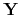-matrix (admittance matrix) gives the relation between input voltages and output currents: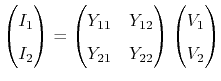(4.82)

with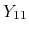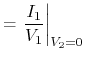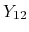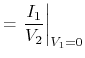(4.83)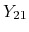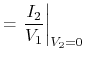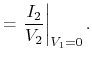(4.84)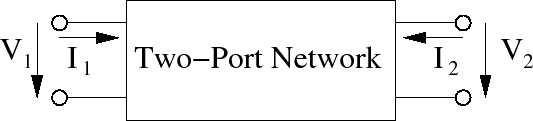Another established relation is the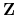-matrix (impedance parameters), which links the output voltages to the input currents: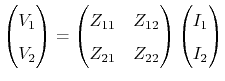(4.85)

with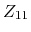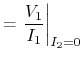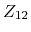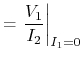(4.86)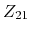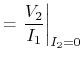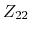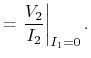(4.87)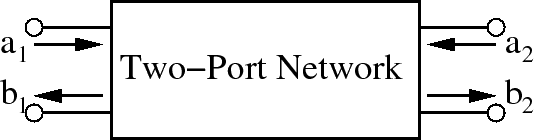However, measurement of those parameter sets requires open or shortcut conditions, which are difficult to achieve at high frequencies. To avoid this problem, matched loads can be used. Thus, the device is embedded into a transmission line with a specific impedance (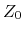). For a traveling wave, the inserted network acts as an impedance, different from the characteristic impedance of the line. S-parameters are the complex valued reflexion and transmission coefficients (Fig. 4.22):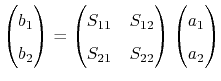(4.88)

with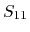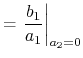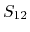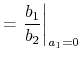(4.89)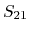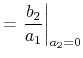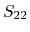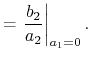(4.90)

The power waves can be expressed as a function of the currents, voltages, and the complex reference impedance .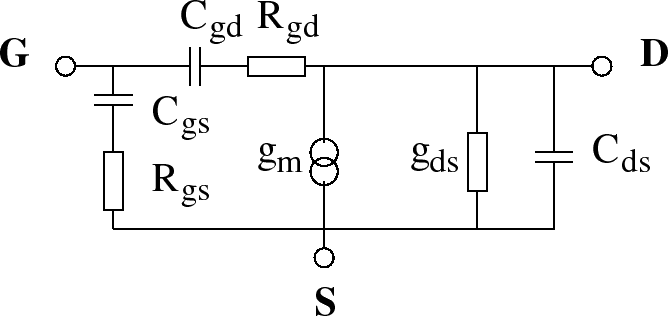In order to obtain important figures of merit for the frequency characteristics of the devices, such as the cut-off frequency and the maximum oscillation frequency, an equivalent circuit is useful. Here, the one used by Dambrine et al.  for FETs is applied (Fig. 4.23). The expressions to calculate the values of its circuit elements are as following [363,364]: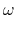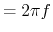(4.91)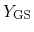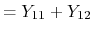(4.92)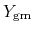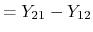(4.93)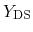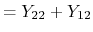(4.94)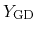(4.95)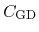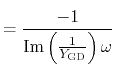(4.96)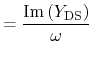(4.97)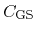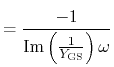(4.98)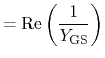(4.99)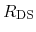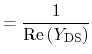(4.100)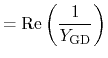(4.101)

As the cut-off frequency is defined as the frequency at which the gain is unity, based on the given equivalent circuit the following expression for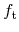can be obtained: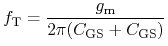with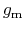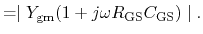In order to separate eventual errors introduced by the measurement equipment it is helpful to embed the device in a parasitic equivalent circuit, independent of the biasing conditions. Such a parasitic equivalent circuit is shown in Fig. 4.24 . The values for those elements are given in Table 4.15.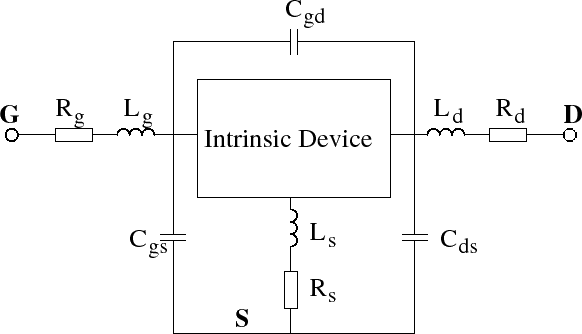Element L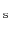L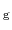L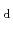C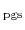C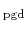C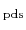Unit [pH] [pH] [pH] [fF] [fF] [fF] Value 1 44 46 18 6 9

S. Vitanov: Simulation of High Electron Mobility Transistors